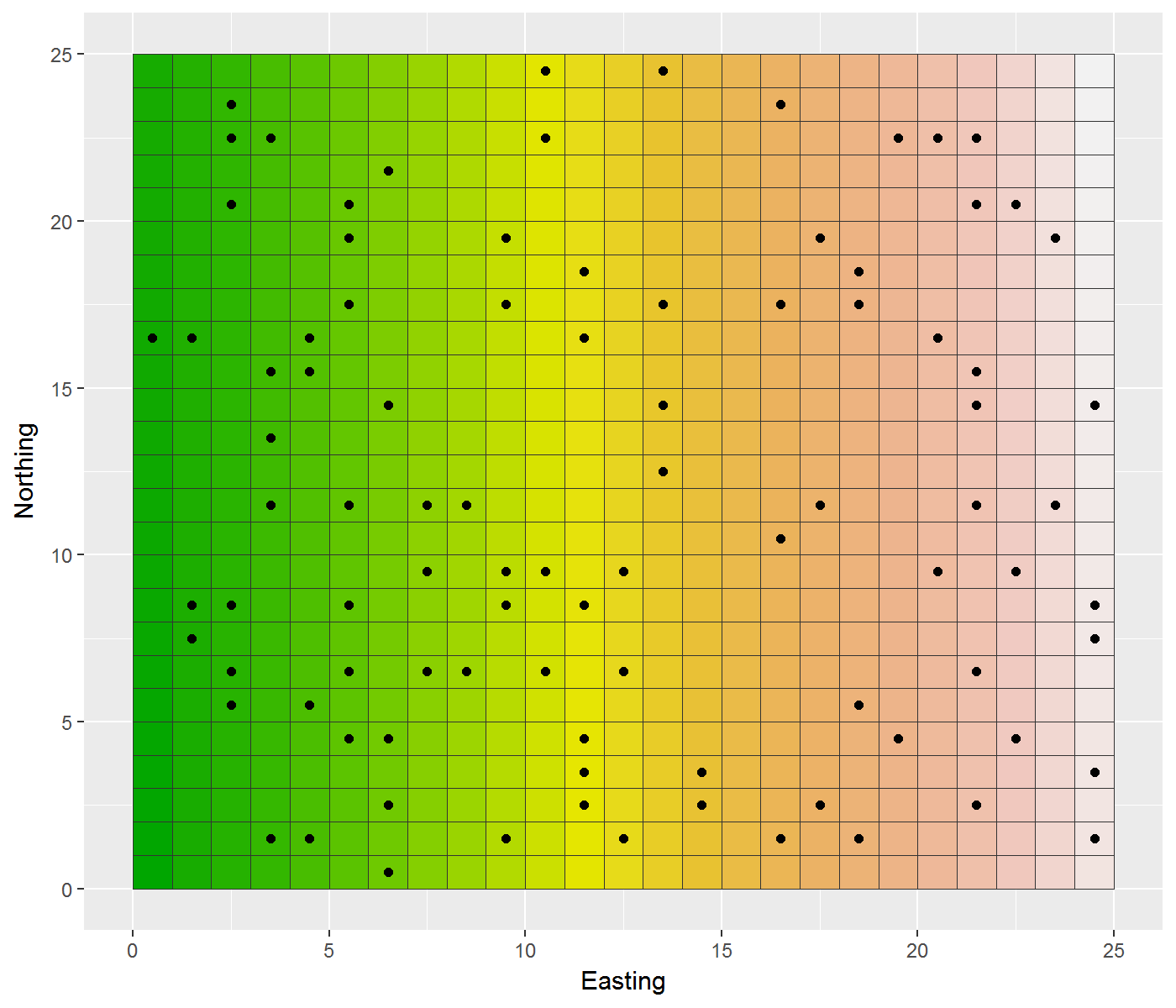# Create random points based on a regular grid

Aim: Create 100 random points based on the cell centre coordinates of a regular grid

Using R, the way we’ll go about it is:

1. Create a grid using the `raster` package to a given extent
2. Extract the cell centre coordinates of each cell
3. Randomly select x,y coordinate pairs until we have the number of samples we want
4. Write them to file

There will then be a bonus level which involves plotting the result where we’ll use `ggplot2`.

``````library(raster)

# Setup some output file names
fout="random_points__CELL_CENTRES.csv"

# Define extent
xmin=0
ymin=0
xmax=25
ymax=25
aoi_extent=extent(xmin, xmax, ymin, ymax)

# Create grid and get xy coordinates from which toi extract these random points)
rast <- raster(ext=aoi_extent, resolution=1)
values(rast)= 1:ncell(rast) # give raster cells a value

# Extract cell centre coordinates
x_centres=xFromCol(rast)
y_centres=yFromRow(rast)

# Select some random points
random_point_count=0
random_point_sample_number=100 # the number of points you want

while (random_point_count < random_point_sample_number){

easting_random=sample(x_centres, 1)
northing_random=sample(y_centres, 1)

if (exists("random_points")==TRUE){
random_points=rbind(random_points,
c("EASTING"=easting_random,
"NORTHING"=northing_random))
} else {
# random_points dataframe doesn't exist yet...")
random_points=data.frame("EASTING"=easting_random,"NORTHING"=northing_random)
}

random_point_count=random_point_count+1
}

# Write random points to file
write.csv(random_points, fout, row.names=FALSE)
``````

Great. Now let’s plot it up - this is based on a couple of stackoverflow posts on the tile plotting here and the coordinate shift here:

``````library(ggplot2)
library(reshape2)

# Setup some output file names
plot_out="random_points__CELL_CENTRES.png"

# Convert raster to matrix
mat=as.matrix(rast)
dat = melt(mat)

# Shift over the easting (now Var1) and northing (now Var2) values by half a pixel (ggplot uses coordinates as mid-points, whereas we want it to plot from the mid-point minus half a pixel length or width)
dat\$Var1=dat\$Var1-0.5
dat\$Var2=dat\$Var2-0.5

p = ggplot(dat, aes(x=Var1, y=Var2)) +
geom_tile(aes(fill=value), colour="grey20") +